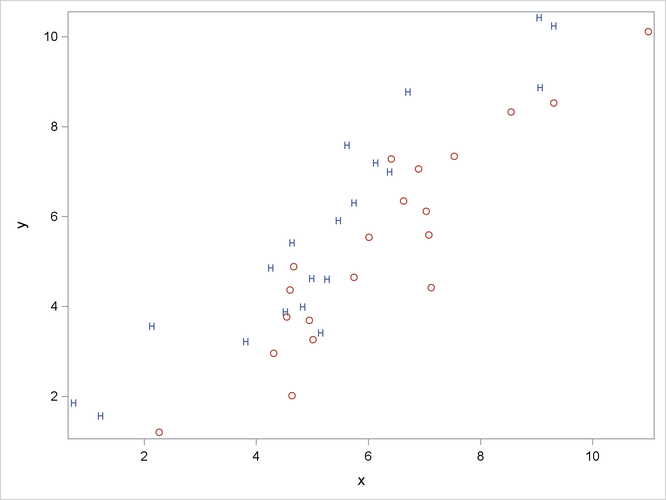## Example: Contrasting Univariate and Multivariate Analyses

Consider an artificial data set with two classes of observations indicated by ‘H’ and ‘O’. The following statements generate and plot the data:

```data random;
drop n;

Group = 'H';
do n = 1 to 20;
x = 4.5 + 2 * normal(57391);
y = x + .5 + normal(57391);
output;
end;

Group = 'O';
do n = 1 to 20;
x = 6.25 + 2 * normal(57391);
y = x - 1 + normal(57391);
output;
end;

run;

proc sgplot noautolegend;
scatter y=y x=x / markerchar=group group=group;
run;
```

The plot is shown in Figure 10.1.

Figure 10.1: Groups for Contrasting Univariate and Multivariate AnalysesThe following statements perform a canonical discriminant analysis and display the results in Figure 10.2:

```proc candisc anova;
class Group;
var x y;
run;
```

Figure 10.2: Contrasting Univariate and Multivariate Analyses

The CANDISC Procedure

 Total Sample Size DF Total 40 39 2 38 2 1

 Number of Observations Read 40 40

Class Level Information
Group Variable
Name
Frequency Weight Proportion
H H 20 20.0000 0.500000
O O 20 20.0000 0.500000

The CANDISC Procedure

Univariate Test Statistics
F Statistics, Num DF=1, Den DF=38
Variable Total
Standard
Deviation
Pooled
Standard
Deviation
Between
Standard
Deviation
R-Square R-Square
/ (1-RSq)
F Value Pr > F
x 2.1776 2.1498 0.6820 0.0503 0.0530 2.01 0.1641
y 2.4215 2.4486 0.2047 0.0037 0.0037 0.14 0.7105

Average R-Square
Unweighted 0.0269868
Weighted by Variance 0.0245201

Multivariate Statistics and Exact F Statistics
S=1 M=0 N=17.5
Statistic Value F Value Num DF Den DF Pr > F
Wilks' Lambda 0.64203704 10.31 2 37 0.0003
Pillai's Trace 0.35796296 10.31 2 37 0.0003
Hotelling-Lawley Trace 0.55754252 10.31 2 37 0.0003
Roy's Greatest Root 0.55754252 10.31 2 37 0.0003

The CANDISC Procedure

Canonical
Correlation
Canonical
Correlation
Approximate
Standard
Error
Squared
Canonical
Correlation
Eigenvalues of Inv(E)*H
= CanRsq/(1-CanRsq)
Test of H0: The canonical correlations in the current row and all that follow are zero
Eigenvalue Difference Proportion Cumulative Likelihood
Ratio
Approximate
F Value
Num DF Den DF Pr > F
1 0.598300 0.589467 0.102808 0.357963 0.5575   1.0000 1.0000 0.64203704 10.31 2 37 0.0003

 Note: The F statistic is exact.

The CANDISC Procedure

Total Canonical Structure
Variable Can1
x -0.374883
y 0.101206

Between Canonical Structure
Variable Can1
x -1.000000
y 1.000000

Pooled Within Canonical Structure
Variable Can1
x -0.308237
y 0.081243

The CANDISC Procedure

Total-Sample Standardized
Canonical Coefficients
Variable Can1
x -2.625596855
y 2.446680169

Pooled Within-Class Standardized
Canonical Coefficients
Variable Can1
x -2.592150014
y 2.474116072

Raw Canonical Coefficients
Variable Can1
x -1.205756217
y 1.010412967

Class Means on Canonical
Variables
Group Can1
H 0.7277811475
O -.7277811475

The univariate R squares are very small, 0.0503 for `x` and 0.0037 for `y`, and neither variable shows a significant difference between the classes at the 0.10 level.

The multivariate test for differences between the classes is significant at the 0.0003 level. Thus, the multivariate analysis has found a highly significant difference, whereas the univariate analyses failed to achieve even the 0.10 level. The raw canonical coefficients for the first canonical variable, `Can1`, show that the classes differ most widely on the linear combination -1.205756217 `x` + 1.010412967 `y` or approximately `y` - 1.2 `x`. The R square between `Can1` and the class variable is 0.357963 as given by the squared canonical correlation, which is much higher than either univariate R square.

In this example, the variables are highly correlated within classes. If the within-class correlation were smaller, there would be greater agreement between the univariate and multivariate analyses.